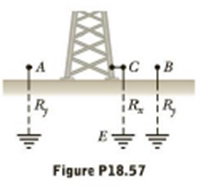Chapter 18, Problem 57AP

Chapter
Section
Textbook Problem

The student engineer of a campus radio station wishes to verify the effectiveness of the lightning rod on the antenna mast (fig. P18.57). the unknown resistance R x is between points C and E. point E is a “true ground” but is inaccessible for direct measurement because the stratum in which it is located is several meters below Earth’s Surface.Two identical rods are driven into the ground at A and B, introducing an unknown resistance R y The procedure for the unknown resistance R x is as follows. Measure resistsnce R 1 between points A and B Then Connect A and B with a heavy conducting wire and measure resistance R 2 between points A and C. (a) Derive a formula For R x in terms of the observable resistances R 1 and R 2 (b) A satisfactory ground resistance would be R x   <2 .0Ω   Is the grounding of the sation adequate if measurements give R 1 =13Ω   and   R 2 =6 .0Ω?To determine
The resistance between the points a and b is, Rab=2717Ω .

Explanation

Given info:

Explanation:

Connect a battery of emf ε between the points a and b.

On applying the Kirchhoff’s rule in the loop acbea,

(1.0)I1(1.0)(I1I3)+ε=0

On re-arranging,

I3=2I1ε (I)

On applying the Kirchhoff’s rule in the loop adbea,

(3.0)I2(5.0)(I2+I3)+ε=0

On re-arranging,

8I2+5I3=ε (II)

On applying the Kirchhoff’s rule in the loop abca,

(3.0)I2+(1.0)I3+(1.0)I1=0

On re-arranging,

I2=I1+I33 (III)

Substitute the equation (I) in (III),

I2=I1+(2I1ε)3=I1ε3 (IV)

Substitute the equations (I) and (IV) in (II),

8(I1ε3)+5(2I1ε

Still sussing out bartleby?

Check out a sample textbook solution.

See a sample solution

The Solution to Your Study Problems

Bartleby provides explanations to thousands of textbook problems written by our experts, many with advanced degrees!

Get Started

Find more solutions based on key concepts